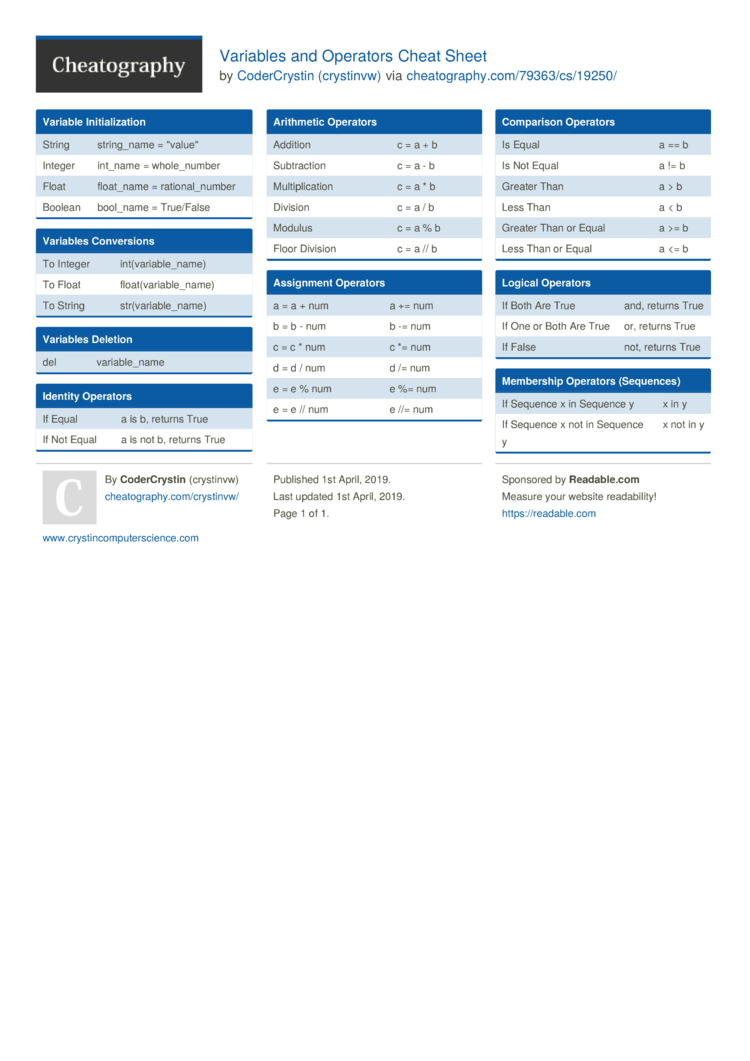# Variables and Operators Cheat Sheet by crystinvw

### Variable Initia­liz­ation

 String string­_name = "­val­ue" Integer int_name = whole_­number Float float_name = ration­al_­number Boolean bool_name = True/False

### Variables Conver­sions

 To Integer int(va­ria­ble­_name) To Float float(­var­iab­le_­name) To String str(va­ria­ble­_name)

### Variables Deletion

 del variab­le_name

### Identity Operators

 If Equal a is b, returns True If Not Equal a is not b, returns True

### Arithmetic Operators

 Addition c = a + b Subtra­ction c = a - b Multip­lic­ation c = a * b Division c = a / b Modulus c = a % b Floor Division c = a // b

### Assignment Operators

 a = a + num a += num b = b - num b -= num c = c * num c *= num d = d / num d /= num e = e % num e %= num e = e // num e //= num

### Comparison Operators

 Is Equal a == b Is Not Equal a != b Greater Than a > b Less Than a < b Greater Than or Equal a >= b Less Than or Equal a <= b

### Logical Operators

 If Both Are True and, returns True If One or Both Are True or, returns True If False not, returns True

### Membership Operators (Seque­nces)

 If Sequence x in Sequence y x in y If Sequence x not in Sequence y x not in y1 Page
//media.cheatography.com/storage/thumb/crystinvw_variables-and-operators.750.jpg

PDF (recommended)